Miscellaneous

Chapter 13 Class 11 Limits and Derivatives
Serial order wise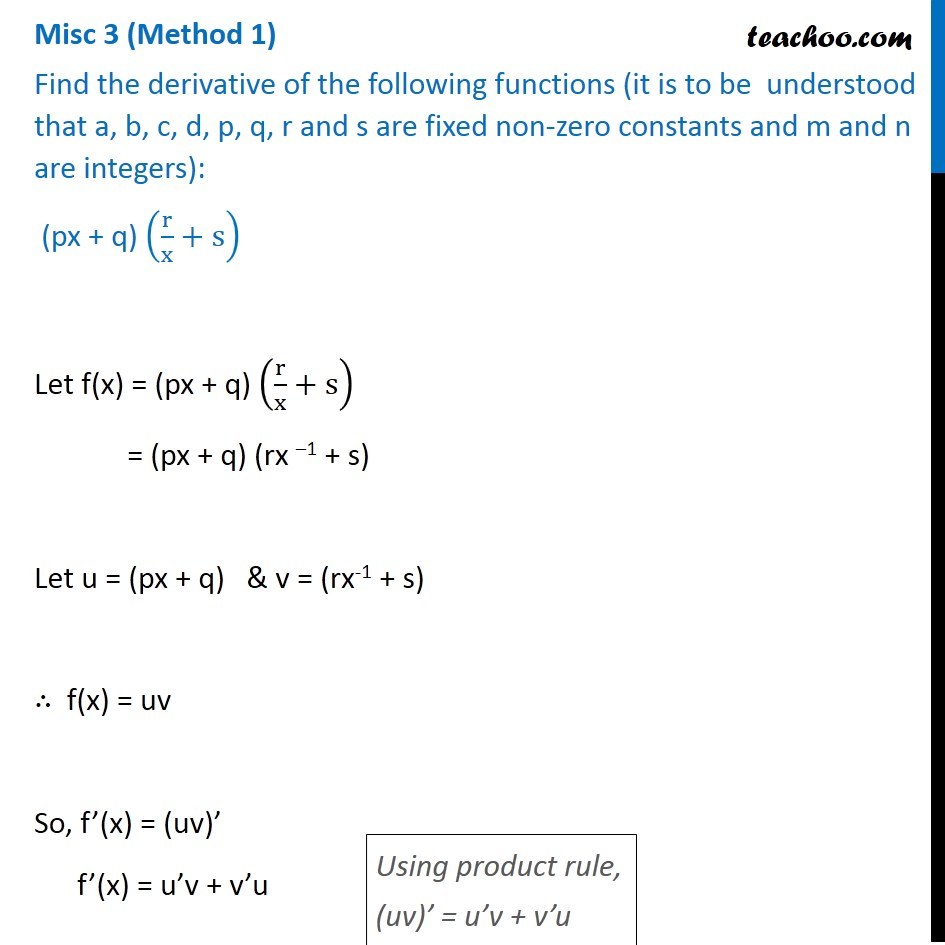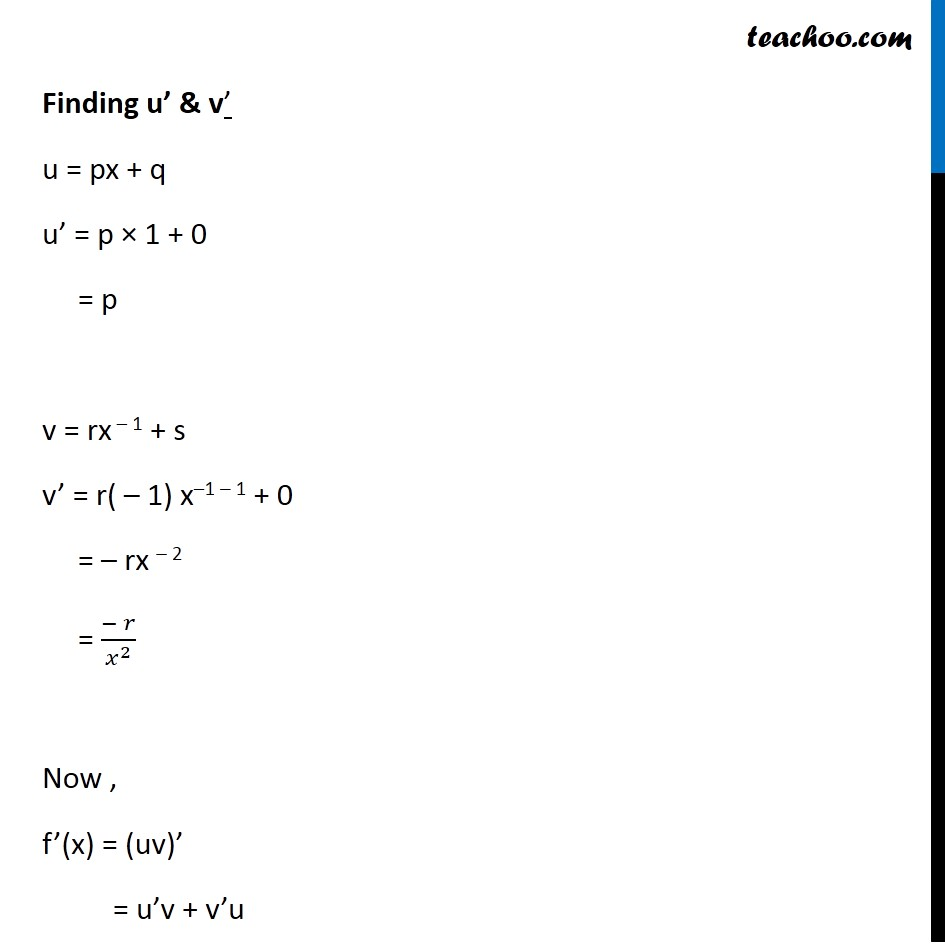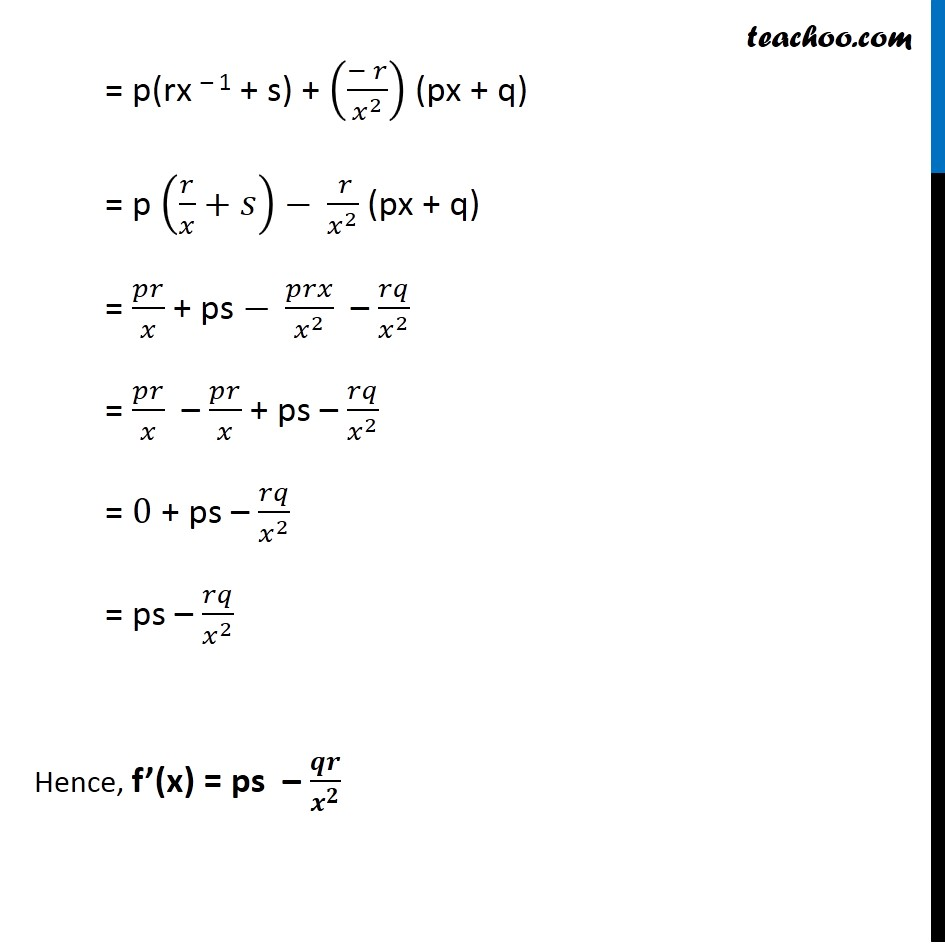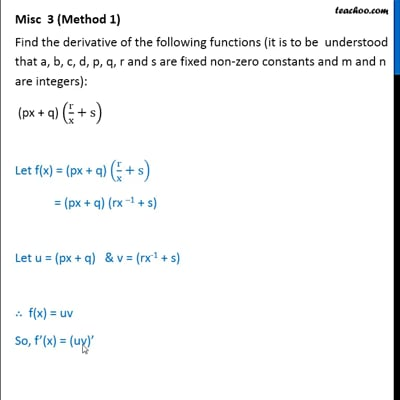This video is only available for Teachoo black users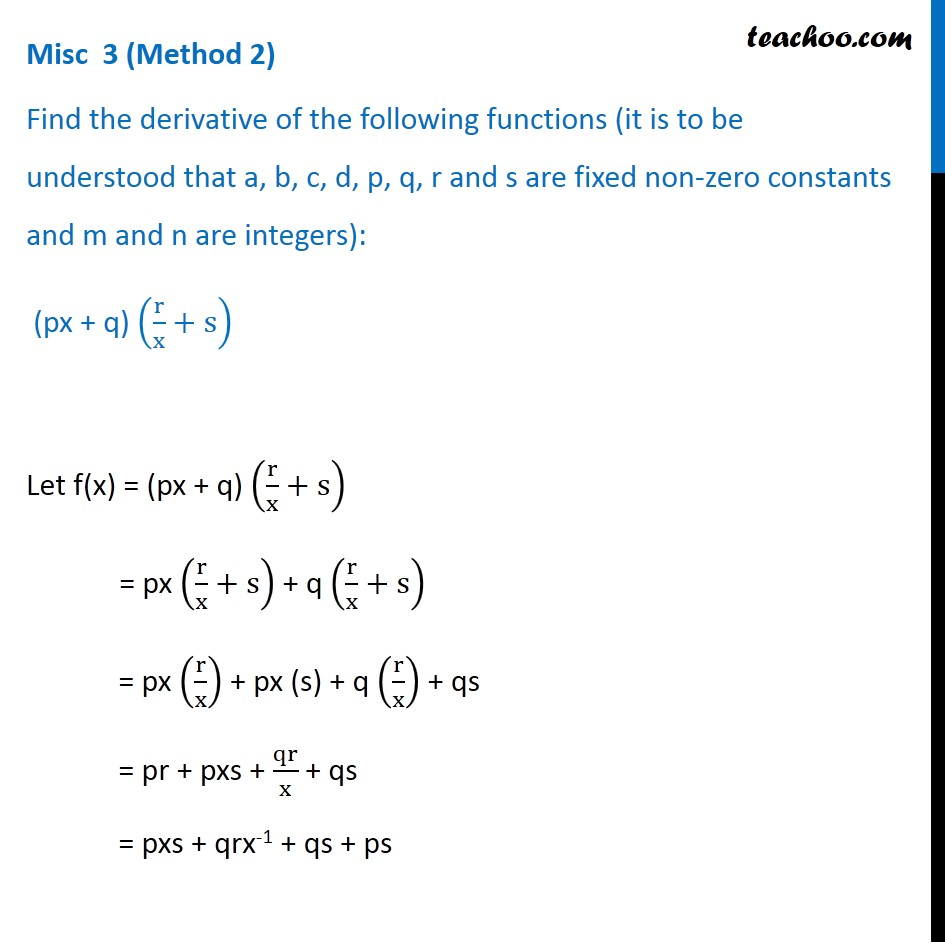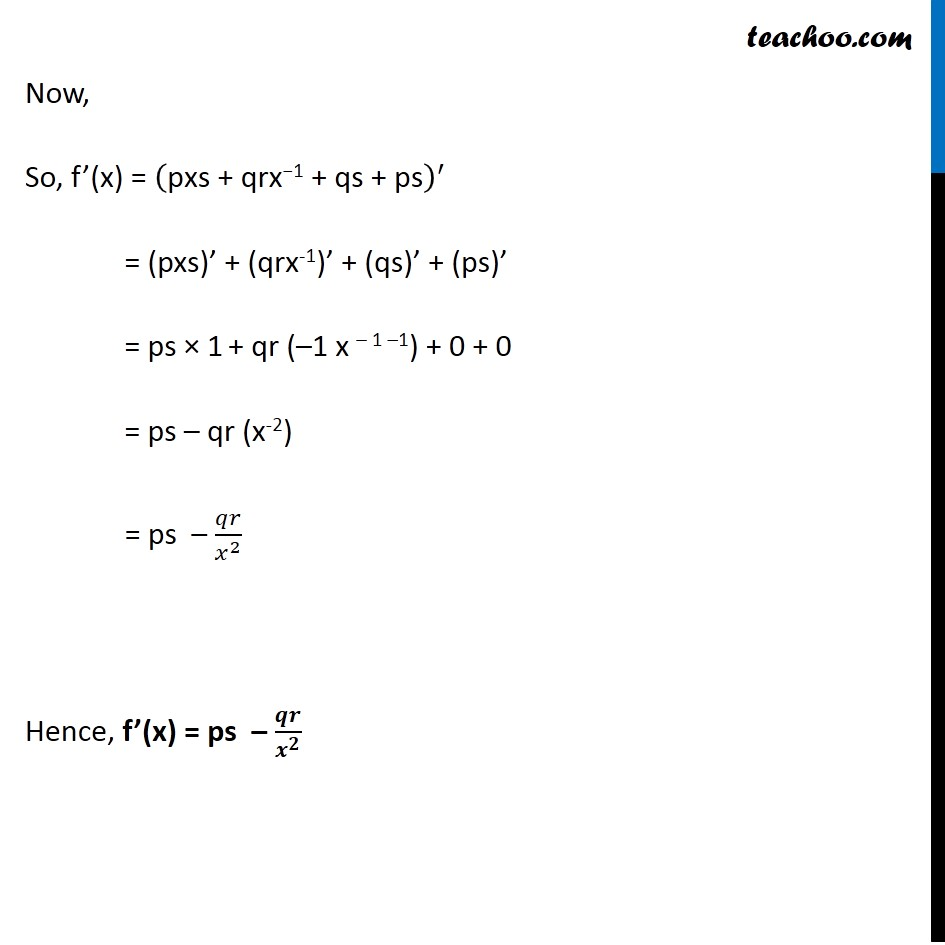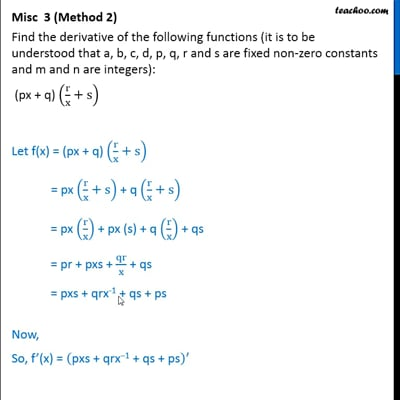This video is only available for Teachoo black users

Solve all your doubts with Teachoo Black (new monthly pack available now!)

### Transcript

Misc 3 (Method 1) Find the derivative of the following functions (it is to be understood that a, b, c, d, p, q, r and s are fixed non-zero constants and m and n are integers): (px + q) (r/x+s) Let f(x) = (px + q) (r/x+s) = (px + q) (rx –1 + s) Let u = (px + q) & v = (rx-1 + s) ∴ f(x) = uv So, f’(x) = (uv)’ f’(x) = u’v + v’u Using product rule, (uv)’ = u’v + v’u Finding u’ & v’ u = px + q u’ = p × 1 + 0 = p v = rx – 1 + s v’ = r( – 1) x–1 – 1 + 0 = – rx – 2 = (− 𝑟)/𝑥^2 Now , f’(x) = (uv)’ = u’v + v’u = p(rx – 1 + s) + ((− 𝑟)/𝑥^2 ) (px + q) = p (𝑟/𝑥+𝑠) − ( 𝑟)/𝑥^2 (px + q) = 𝑝𝑟/𝑥 + ps − 𝑝𝑟𝑥/𝑥^2 – 𝑟𝑞/𝑥^2 = 𝑝𝑟/𝑥 – 𝑝𝑟/𝑥 + ps – 𝑟𝑞/𝑥^2 = 0 + ps – 𝑟𝑞/𝑥^2 = ps – 𝑟𝑞/𝑥^2 Hence, f’(x) = ps – 𝒒𝒓/𝒙^𝟐 Misc 3 (Method 2) Find the derivative of the following functions (it is to be understood that a, b, c, d, p, q, r and s are fixed non-zero constants and m and n are integers): (px + q) (r/x+s) Let f(x) = (px + q) (r/x+s) = px (r/x+s) + q (r/x+s) = px (r/x) + px (s) + q (r/x) + qs = pr + pxs + qr/x + qs = pxs + qrx-1 + qs + ps Now, So, f’(x) = ("pxs + qrx−1 + qs + ps" )^′ = (pxs)’ + (qrx-1)’ + (qs)’ + (ps)’ = ps × 1 + qr (–1 x – 1 –1) + 0 + 0 = ps – qr (x-2) = ps – 𝑞𝑟/𝑥^2 Hence, f’(x) = ps – 𝒒𝒓/𝒙^𝟐